x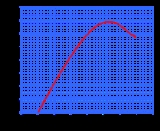Lift coefficientEncyclopedia
The lift coefficient is a dimensionless coefficient that relates the lift
Lift (force)
A fluid flowing past the surface of a body exerts a surface force on it. Lift is the component of this force that is perpendicular to the oncoming flow direction. It contrasts with the drag force, which is the component of the surface force parallel to the flow direction...

generated by a lift
Lift
Lift may mean:* Lift , a mechanical force generated by an object moving through a fluid* Lift , rising air used by soaring birds and glider, hang glider and paraglider pilots for soaring flight...

ing body, the dynamic pressure of the fluid flow around the body, and a reference area associated with the body. A lifting body is a foil
Foil (fluid mechanics)
A foil is a solid object with a shape such that when placed in a moving fluid at a suitable angle of attack the lift is substantially larger than the drag...

or a complete foil-bearing body such as a fixed-wing aircraft
Fixed-wing aircraft
A fixed-wing aircraft is an aircraft capable of flight using wings that generate lift due to the vehicle's forward airspeed. Fixed-wing aircraft are distinct from rotary-wing aircraft in which wings rotate about a fixed mast and ornithopters in which lift is generated by flapping wings.A powered...

.

Lift coefficient is also used to refer to the dynamic lift characteristics of a two-dimensional foil section, whereby the reference area is taken as the foil chord
Chord (aircraft)
In aeronautics, chord refers to the imaginary straight line joining the trailing edge and the center of curvature of the leading edge of the cross-section of an airfoil...

.

Lift coefficient may be described as the ratio of lift pressure to dynamic pressure where lift pressure is the ratio of lift to reference area.

Lift coefficient may be used to relate the total lift
Lift (force)
A fluid flowing past the surface of a body exerts a surface force on it. Lift is the component of this force that is perpendicular to the oncoming flow direction. It contrasts with the drag force, which is the component of the surface force parallel to the flow direction...

generated by a foil-equipped craft to the total area of the foil. In this application the lift coefficient is called the aircraft or planform
Planform
In aviation, a planform is the shape and layout of a fixed-wing aircraft's fuselage and wing. Of all the myriad planforms used, they can typically be grouped into those used for low-speed flight, found on general aviation aircraft, and those used for high-speed flight, found on many military...

lift coefficient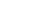Watercraft and automobiles equipped with fixed foils can also be assigned a lift coefficient.

The lift coefficient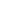is equal to: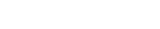where
•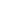is the lift force
Lift (force)
A fluid flowing past the surface of a body exerts a surface force on it. Lift is the component of this force that is perpendicular to the oncoming flow direction. It contrasts with the drag force, which is the component of the surface force parallel to the flow direction...

,
•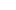is fluid
Fluid
In physics, a fluid is a substance that continually deforms under an applied shear stress. Fluids are a subset of the phases of matter and include liquids, gases, plasmas and, to some extent, plastic solids....

density
Density
The mass density or density of a material is defined as its mass per unit volume. The symbol most often used for density is ρ . In some cases , density is also defined as its weight per unit volume; although, this quantity is more properly called specific weight...

,
•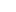is true airspeed
True airspeed
True airspeed of an aircraft is the speed of the aircraft relative to the airmass in which it is flying. True airspeed is important information for accurate navigation of an aircraft.-Performance:...

,(speed of the body relative to a static point on the earth's surface)
•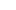is dynamic pressure, and
•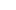is planform
Planform
In aviation, a planform is the shape and layout of a fixed-wing aircraft's fuselage and wing. Of all the myriad planforms used, they can typically be grouped into those used for low-speed flight, found on general aviation aircraft, and those used for high-speed flight, found on many military...

area.

The lift coefficient is a dimensionless number.

The aircraft lift coefficient can be approximated using the Lifting-line theory
Lifting-line theory
Lifting-line theory or Lanchester-Prandtl wing theory was published by Ludwig Prandtl in 1918–1919 after working with Albert Betz and Max Munk on the problem of a useful mathematical tool for examining lift from "real world" wings....

or measured in a wind tunnel
Wind tunnel
A wind tunnel is a research tool used in aerodynamic research to study the effects of air moving past solid objects.-Theory of operation:Wind tunnels were first proposed as a means of studying vehicles in free flight...

test of a complete aircraft configuration.

## Section lift coefficient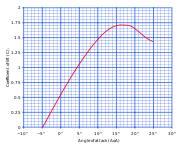Lift coefficient may also be used as a characteristic of a particular shape (or cross-section) of an airfoil
Airfoil
An airfoil or aerofoil is the shape of a wing or blade or sail as seen in cross-section....

. In this application it is called the section lift coefficient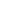It is common to show, for a particular airfoil section, the relationship between section lift coefficient and angle of attack
Angle of attack
Angle of attack is a term used in fluid dynamics to describe the angle between a reference line on a lifting body and the vector representing the relative motion between the lifting body and the fluid through which it is moving...

. It is also useful to show the relationship between section lift coefficient and drag coefficient
Drag coefficient
In fluid dynamics, the drag coefficient is a dimensionless quantity that is used to quantify the drag or resistance of an object in a fluid environment such as air or water. It is used in the drag equation, where a lower drag coefficient indicates the object will have less aerodynamic or...

.

The section lift coefficient is based on two-dimensional flow - the concept of a wing with infinite span and non-varying cross-section, the lift of which is bereft of any three-dimensional effects. It is not relevant to define the section lift coefficient in terms of total lift and total area because they are infinitely large. Rather, the lift is defined per unit span of the wing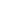In such a situation, the above formula becomes: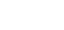whereis the chord
Chord (aircraft)
In aeronautics, chord refers to the imaginary straight line joining the trailing edge and the center of curvature of the leading edge of the cross-section of an airfoil...

length of the airfoil.

The section lift coefficient for a given angle of attack can be approximated using the thin airfoil theory, or determined from wind tunnel tests on a finite-length test piece, with end-plates designed to ameliorate the three-dimensional effects associated with the trailing vortex wake structure.

Note that the lift equation does not include terms for angle of attack
Angle of attack
Angle of attack is a term used in fluid dynamics to describe the angle between a reference line on a lifting body and the vector representing the relative motion between the lifting body and the fluid through which it is moving...

— that is because the mathematical relationship between lift
Lift (force)
A fluid flowing past the surface of a body exerts a surface force on it. Lift is the component of this force that is perpendicular to the oncoming flow direction. It contrasts with the drag force, which is the component of the surface force parallel to the flow direction...

and angle of attack
Angle of attack
Angle of attack is a term used in fluid dynamics to describe the angle between a reference line on a lifting body and the vector representing the relative motion between the lifting body and the fluid through which it is moving...

varies greatly between airfoils and is, therefore, not constant. (In contrast, there is a straight-line relationship between lift and dynamic pressure; and between lift and area.) The relationship between the lift coefficient and angle of attack is complex and can only be determined by experimentation or complicated analysis. See the accompanying graph. The graph for section lift coefficient vs. angle of attack follows the same general shape for all airfoils
Airfoil
An airfoil or aerofoil is the shape of a wing or blade or sail as seen in cross-section....

, but the particular numbers will vary. The graph shows an almost linear increase in lift coefficient with increasing angle of attack
Angle of attack
Angle of attack is a term used in fluid dynamics to describe the angle between a reference line on a lifting body and the vector representing the relative motion between the lifting body and the fluid through which it is moving...

, up to a maximum point, after which the lift coefficient reduces. The angle at which maximum lift coefficient occurs is the stall
Stall (flight)
In fluid dynamics, a stall is a reduction in the lift coefficient generated by a foil as angle of attack increases. This occurs when the critical angle of attack of the foil is exceeded...

angle of the airfoil.

The lift coefficient is a dimensionless number.

Note that in the graph here, there is still a small but positive lift coefficient with angles of attack less than zero. This is true of any airfoil with camber
Camber (aerodynamics)
Camber, in aeronautics and aeronautical engineering, is the asymmetry between the top and the bottom surfaces of an aerofoil. An aerofoil that is not cambered is called a symmetric aerofoil...

(asymmetrical airfoils). On a cambered airfoil at zero angle of attack the pressures on the upper surface are lower than on the lower surface.

## See also

• Fluid
Fluid
In physics, a fluid is a substance that continually deforms under an applied shear stress. Fluids are a subset of the phases of matter and include liquids, gases, plasmas and, to some extent, plastic solids....

• Density
Density
The mass density or density of a material is defined as its mass per unit volume. The symbol most often used for density is ρ . In some cases , density is also defined as its weight per unit volume; although, this quantity is more properly called specific weight...

• Foil (fluid mechanics)
Foil (fluid mechanics)
A foil is a solid object with a shape such that when placed in a moving fluid at a suitable angle of attack the lift is substantially larger than the drag...

• Drag coefficient
Drag coefficient
In fluid dynamics, the drag coefficient is a dimensionless quantity that is used to quantify the drag or resistance of an object in a fluid environment such as air or water. It is used in the drag equation, where a lower drag coefficient indicates the object will have less aerodynamic or...

• Pitching moment
Pitching moment
In aerodynamics, the pitching moment on an airfoil is the moment produced by the aerodynamic force on the airfoil if that aerodynamic force is considered to be applied, not at the center of pressure, but at the aerodynamic center of the airfoil...

• Circulation control wing
Circulation control wing
A circulation control wing is a form of high-lift device for use on the main wing of an aircraft to increase the lift coefficient. CCW technology has been in the research and development phase for over sixty years, and the early models were called blown flaps.The CCW works by increasing the...

• Zero lift axis
Zero lift axis
When symmetric aerofoils are moving parallel to the chord line of the aerofoil, zero lift is generated. However, when cambered aerofoils are moving parallel to the chord line, lift is generated...

The source of this article is wikipedia, the free encyclopedia.  The text of this article is licensed under the GFDL.